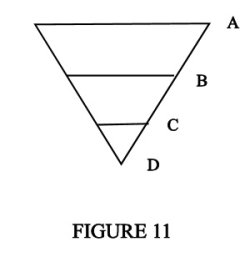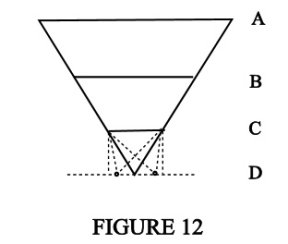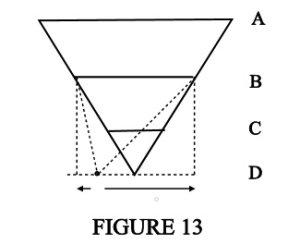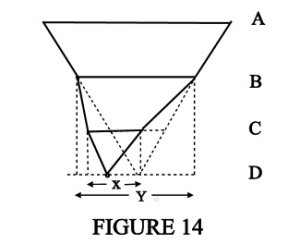THE FRACTAL MATRIX:

(Sept., 2004)

PART V

By Noel Huntley, Ph.D.

In this section we shall concentrate on the fractal relations, randomness, and free will for the general and 'big picture': Source to particles.

Consider Figure 11, which is like an inverted pyramid. Let us first try to understand the relationship between this diagram with its upside-down triangle, and other diagrams in which the source, or highest level of control, such as a president, is depicted by a point, or small circle (figures 4 and 8, Part I and III). However, we know that this source spans everything below it---a president integrates a whole company, or a God is the source of all things. Thus in Figure 11 the top horizontal line depicts this complete span (of everything below this level). The point D is one of many such points at this level.Now as we have discussed, energies are fundamentally holographic; the whole A is expressed in reducing states B, C, D, etc., down to a particle, say, D. The dimensions and particle level at D might correspond to our 3D existence. The level C would be a higher fractal involving higher dimensions. That is, D does not know about C, B, A, at least externally. Note that these inverted triangle figures also depict the vortex spiral described in previous articles.

Imagine now the part D, such as a particle or large living entity or fractal of consciousness, expressing an existence, say, simply by moving about. If we make the structure A to D, that is, the whole inverted pyramid, a rigid one, and we lock together the fractal levels A, B, C, D, this means that level B is run entirely by the source A; level C is run by B; and D by C, which means A controls D. Or, in other words, there is a continuous and direct control from A to D; it is one fixed, whole, or entity---the source expressing through D. But everything is known in this set-up. The source spans the entire activities, or even the life of the 'particle' D, all at once. The particle D totally obeys A. It has no existence of its own. The source also knows exactly the outcome of everything it does with D. Or, D either, similarly, knows its future, if it is given direct access to A, if not, and at the other extreme, it is totally an unaware puppet under the control of A. Entity A is simply being D, that is, A's expression in the lower dimension.

In the above example, D has no free will (at D). Let us see now how fractals enter into this configuration manifesting free will or choice.

Our inverted pyramid is divided into fractal levels A, B, C, D. There could be any number. This number determines the attributes of existence and is part of the design of the universe. In Figure 12 we have at C the first fractal level which determines what happens at D. The length of the horizontal line at C is a measure of the free will, or degree of choices. This is also the amount of variety and also potential randomness and unpredictability. Thus at D the degree of freedom, or 'distance', can't be more than that governed by the horizontal line length at C. This gives an idea of the degree of free will that C can express at level D. If we wish, we could make C the human mind and consciousness, and D the body and material events. Thus the first fractal level is then really C to D.We now apply the same procedure to fractal level B---Figure 13. We see that the horizontal is wider and therefore there is more space, more freedom and more choice amongst greater potential probabilities. But of course we can't actually take these levels separately. They work together; Figure 12 and 13 must be combined. Figure 14 shows this relationship. Note that it is the same principle as given earlier in Parts I - IV of the person taking the dog for a walk, using a long leash so that the dog has freedom to move around but the main overall control comes from the person.In Figure 14, the freedom along X of fractal C is superimposed by the freedom of fractal B along Y. That is, Y is carrying X; these are merely two (only) interacting variables. In the figure we can next also consider the source level A in the same way. Thus in this simple example, we would have three variables, levels A, B, C, acting simultaneously to produce the resultant behaviour at D. Note that this same structural pattern occurs with knowledge and contexts, as discussed in other articles, for example, The Limits of Scientific Objectivity. The fractal C to D, which doesn't know about B, thinks its knowledge is based on the (apparent) closed system CD. That is, thinks C is relative to a zero---that B does not exist, and therefore C is absolute, or a constant. But C is open to B, and is thus a higher-order open system, and needs to be eventually recognised for proper evolution of CD to take place. In our society everything is directed at keeping the system CD closed.

Note that the upright pyramid, with a single point at the top and representing the whole of everything below, is the objective format (with current science, unity needs to be a point---one state) and the figure here, the inverted pyramid, is the subjective one, and more realistic for our discussion in this case.

We see that the fractal levels break up the control from A, enabling proportionate control to be exercised by the lower levels, B and C in this example. We can see that the future for D is now unknown since it is being carried not only by the energies of C, but, in turn, by B; and B by A. Thus A can set itself not to know the outcome by letting go of B and C, but A could intervene at any of these levels; just as one may move a finger (level D), say, down, and the arm (level A) override it by moving up.

Note that closed systems can be formed at any of the fractal levels---there may be any number of fractals between D and A. If consciousness is aware of being in a closed system, then that consciousness is also looking from outside this system---otherwise it would not be aware of even the boundary. However, the ego's very nature is to form closed systems. It will reject the higher fractals, such as 'soul'. This is a threat to its very structure, of which the latter emphasises the ego self (lower fractal human level).

The ego will also fix boundaries around knowledge (then take this boundary as a zero). If it formulates, say, Newton's laws, or learns them, as implied, it will insist they cover all phenomena, and not consider that the laws could be part of a larger integration (in which maybe the laws can be bypassed, or certain processes of nature don't use them).

Remember that the geometric relationship in, for example, Figure 14, such as the lines X and Y, and that X is 'carried' by Y, is conceptual. Level Y can override the thinking of X. Level X cannot think beyond C. X will think it is totally in control unless it has done a lot of 'philosophical' thinking and/or is highly intuitive. The latter will enable it to sense the higher fractals of the total self. In case the reader spots the projection here but is unfamiliar with it; yes, it does mean everyone is God/source at level A. Apparently there is only one 'self' (which note, gives the greatest symmetry re tests of truth in physics).

Now, the human at CD can create his or her own programmes. But supposing they are overridden and contradicted by the soul level, which will be B in this configuration, since we let C be the human mind/consciousness. If there is a sufficient gap (equals blockage) between CD and BC (compare longer dog's leash), then CD can take a negative path (opposing the soul programme BC) since the ego---a closed system---is now stronger (more independent). Nevertheless, there is still a 'karma' mechanism in which the energy splits into a polarity. One pole 'allows' and expresses the negativity but the other pole eventually returns the flow in an attempt to rebalance and restore the duality to unity---like phase conjugation (see The Mechanics of Karma).

The model (Figures 11 to 14), we have described here is the 'big picture' ranging from the whole to a mere point. Now this same system, describing mainly the way consciousness works can also be used to illustrate the geometric features of nature's (mind/universal) computer and information system. The only way a geometric system can work successfully is through the fractal gradient, culminating in one whole---a region that spans, simultaneously, all its parts.

Any appropriate region of nature selected for analysis as an informational system will have the fractal configuration of Figure 14. One energy or quantum state will span the whole region but will have a breakdown (quantum reduction) through sub-fractals down to the basic element manifesting in 3D, or simply manifesting the action as in skills. For example, an arm movement has programmes at the joints, shoulder, elbow, and hand. But the shoulder contains the whole programme and passes down (quantum reduces) its appropriate components to the elbow, and hand (see other articles on fractals and physical mobility).

The computer programmes of nature must utilise this fractal gradient. The reason is simply because this is how energy and creation appears in 3D. Further, these programmes are not detached from the Source, for example, A in Figure 14. The number of fractal levels depends on the application, for example, a tree has about seven levels on the average; the arm, four principal levels at the joints (fingers, wrist, elbow, shoulder); consciousness, in its native state and in theory, would have a complete and continuous gradient---would have infinite fractal and holographic properties.

The important feature of the programme system is to be able to locate a particle in spacetime or to position one precisely in spacetime. The growth of organisms depends on this; also a universe will have all its parts interrelated in this fashion so that every part is known in relationship to all other parts---the holographic effect.

In Figure 14 we have programmable levels A, B, C, D; for example, in skills: shoulder (A), elbow (B), wrist (C), and fingers (D). Let the horizontals at A, B, C, give the programme ranges. Note that, as explained previously, although the energies of D, C, B, A are 'nested', that is within one another (going into inner space, higher dimensions, from D to A) in the physical arm (and tree, etc.) they project out linearly at the joints: fingers, wrist, elbow and shoulder.

Now if we fix (embed, programme) a point on these horizontals, for example in Figure 14, the dot at D has a particular position on the horizontal that determines position of the vertex of the inverted pyramid, then do this with the upper pyramid sections, this would constitute a programme that interrelates A, B, C, D and which could determine the angle of the joints at the shoulder, elbow, wrist, and fingers. As mentioned, nature's programmes work on geometry; the position of limbs in coordination will have a coding system that directly relates their spatial and temporal geometric relations. The above, however, is merely a superficial reference to fractal applications in programming.